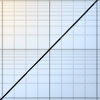#### You may also like### Integral Equation

Solve this integral equation.### Integral Sandwich

Generalise this inequality involving integrals.### Integral Inequality

An inequality involving integrals of squares of functions.

# Area L

### Why do this problem?

This problem looks more complicated than it is. Students need to interpret the notation and use their understanding of definite integrals as areas under curves to prove a formula graphically. Once they make sense of the formula in terms of a diagram, they can use it to evaluate integrals which might prove challenging to calculate by other means.

### Possible approach

Students could sketch a suitable continuous increasing function on paper or whiteboards, and mark on suitable points $a, b, f(a)$ and $f(b)$. Give them time in groups to identify the areas on their graph represented by $\int_{f(a)}^{f(b)} f^{-1}(t) \,dt$, $\int_a^bf(x) \,dx$, $bf(b)$ and $af(a)$.

Once they are convinced that the proof of the formula follows from a diagram, give them the chance to verify the formula for cases they can integrate directly, like $\int_a^b \sqrt{t} \,dt$.

The problem suggests using the formula to evaluate $\int_0^1 \sin^{-1}t \,dt$ but other inverse functions of continuous increasing functions can be used, and it offers good integration practice to allow students to suggest functions of their own to integrate which they can evaluate by other means as a check. It is worth taking the time to discuss the requirement for the function to be increasing between $a$ and $b$, and setting the challenge to find a similar formula for a function which is decreasing.

### Key questions

On the graph $y=f(x)$, what is represented by $\int_{f(a)}^{f(b)} f^{-1}(t) \,dt$?
Why does the formula specify that $f(x)$ is increasing?
What could be done if $f(x)$ were decreasing?

### Possible extension

Integral Sandwich is another problem where sketching a graph makes the meaning of the integrals much clearer.

### Possible support

Sketching some particular inverse functions like $y=\sqrt x$ and marking on numerical values for a and b, then working out the relevant areas might provide a way into this problem.